Problems & Puzzles: Problems

Problem 38.  Sebastián Martín Ruiz- Prime formulas

Sebastián Martín Ruiz, a high school teacher from Chipiona, Cadiz, Spain, has came up  with his own prime formulas p(n) and nxt(p)

• p(n) calculates the n-th prime number. See :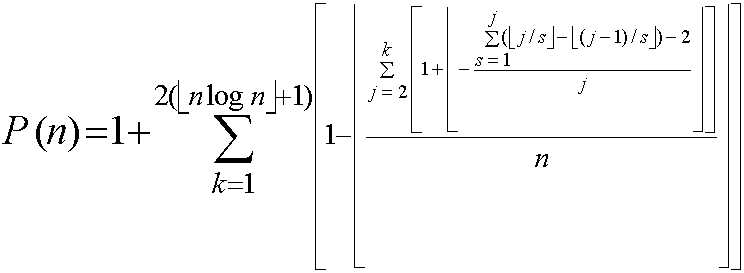(here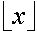means the largest integer less than or equal to x; in Ubasic this corresponds to the function "int(x)" or "floor(x)")

• nxt(p) calculate the following prime to another given p. See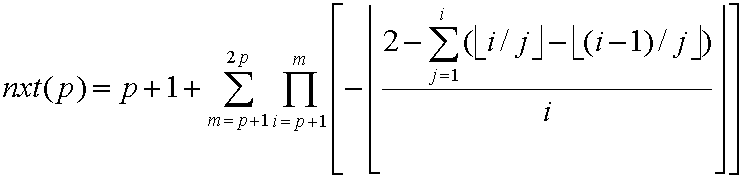Sebastian does not claim that his formulas satisfy one of the desiderata asked by Hardy and Wright for these mathematical targets:

"Is there a simple general formula for the n-th prime pn (a formula, that is to say, by which we can calculate the value of pn for any given n with less labour than by the use of the sieve of Eratosthenes)?"

because he recognizes that both functions should work slower than the corresponding algorithms based in the sieve of Erathostenes.

(This happens due to the very nature of one concept at the core of both formulas: the exhaustive calculation of the number of divisors of a number n; this calculation is needed to evaluate the so-called Smarandache Prime function, a binary one, that constitutes - at the end - the essential calculation)

Nevertheless - with this feature against - Sebastián hopes indeed that  at least his formulas are better than the previously produced by others - like Sierpinski, Willans & Gandhi. See   - in two specific aspects:

• His formulas do not suppose any previous knowledge or calculation of any prime number.
• His formulas are "faster" than any other formulas alike in order to make the same calculations.

As a matter of fact Sebastián claims that both formulas are of a polynomial order - k*(n log n)^3 - while the previous ones are factorial or exponential order.

Questions:

1) Do you agree with the Sebastian's  two before claims?

In the Ribenboim's interpretation of the Hardy & Wright desiderata for the prime formula he says:

"What is intended... is to find a closed expression for the n-th prime pn in terms of n, by means of functions that are computable and , if possible, classical" (p.179, op. cit.)

2) Dou you think that the Sebastian's formulas are in terms of n, closed, computable and classical?

3) From a computational point of view, are the Sebastian's prime formulas an improvement or a back step regarding the Liu Fenghsui' s prime formula? (See Problem 37 in these pages)

4) Do you devise any way to optimize the Sebastian's prime formulas?

_________________
Notes:

: Sebastián Martín Ruiz original publications can be found here: p(n) and nxt(p). The formulas were developed in 1999 but published in the SJN at Spring 2000. At the time of the publications he was considering to be the only author of the main inner functions [ d(n) and G(n) ]. Only recently he found that d(n) was already developed, according to "Introducción al la teoría de números", Ivan Niven & H. S. Zuckerman. Editorial Limusa-Wiley, S.A. México, 1969. pp. 88-89-90.

 & : Here are my Ubasic-codes to calculate these functions, and below the Sebastian's ones for Mathematica.

 p(n) nxt(p) 10 input N:clr time:'pnsmr.ub 20 S1=0:Z=2*(int(N*log(N))+1) 30 for K=1 to Z 40 S2=0 50 for J=2 to K 60 S3=0 70 for L=1 to J 80 S3=S3+J\L-(J-1)\L 90 next L 100 S2=S2+1+(2-S3)\J 110 next J 120 S1=S1+1-S2\N 130 next K 140 print "The prime";N;"-th is=";S1+1; 150 if S1+1<>prm(N) then print "Error":end 160 print "Verified y Right",time:end 10 input P:clr time:'pqsmr.ub 20 S1=0 30 for M=P+1 to 2*P 40 F=1 50 for I=P+1 to M:S2=0 60 for J=1 to I:S2=S2+I\J-(I-1)\J:next J 70 S2=2-S2:F=F*(-(S2\I)) 80 next I 90 S1=S1+F 100 next M 110 print "nxt(";P;")=";P+1+S1,time:end DD[i_]:=Sum[Floor[i/k]-Floor[(i-1)/k],{k,1,i}] FG[n_]:=Sum[1+Floor[-(DD[j]-2)/j],{j,2,n}] VIC[n_]:=1+Sum[1-Floor[FG[k]/n], {k,1,2*(Floor[n*N[Log[n],15]]+1)}] Do[Print[VIC[n]," ",Prime[n]],{n,1,25}] p=Input["Input a prime number:"] DD[i_]:=Sum[Floor[i/j]-Floor[(i-1)/j],{j,1,i}] G[i_]:=-Floor[(2-DD[i])/i] F[m_]:=Product[G[i],{i,p+1,m}] S[n_]:=Sum[F[m],{m,n+1,2*n}] Print["nxt(",p,")=",p+1+S[p]]

 P. Ribenboim, The New Book of Prime Number Records, pp. 179-186

Solution:

Jud McCranie has suggested to eliminate the 'floor' or the 'int' functions in the codes that I  originally wrote in Ubasic, using - instead - the integer division operator  (x\y). I have followed his suggestion and the savings in time of computation has been of .... 33.3%... nothing bad!

Now the codes shown in the table above reflect that idea.

***

The Jud's idea also can be applied to the formulas writing, eliminating the clumsy floor brackets so hard to write in a basic word processor... And I would like if that idea can be implemented also in the codes for Mathematica, with the same good results in time savings that we got in Ubasic

***

I (CR) have devised another important improvement in the codes based in the fact that the calculation of the number of divisors of a number n, exhaustive via, needs run only from 1 to isqrt(n) and then multiply for 2 the result obtained. The changes needed in my codes are:

 Function Changes in red p(n) 70 for L=1 to isqrt(J) 100 S2=S2+1+(2-2*S3)\J nxt(p) 60 for J=1 to isqrt(I):S2=S2+I\J-(I-1)\J:next J 70 S2=2-2*S2:F=F*(-(S2\I))

The times spent in calculation drop dramatically!

BTW nxt(p): while nxt(p) is the following prime to the number p, p itself needs not to be prime!

***

The Sebastian's formulas introducing the ideas given by Jud and me (CR), and homogenizing the sub-indexes in both formulas, look the following way: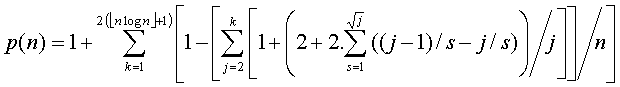and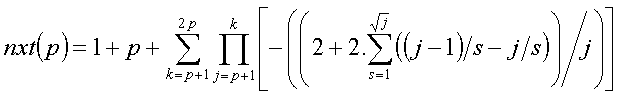in these formulas all the divisions are integer-divisions

***

The new programs for Mathematica, sent by Sebastián, are:

 Function Program p(n) DD[i_]:=Sum[Quotient[i,k]-Quotient[(i-1),k],{k,1,Floor[Sqrt[i]]}] FG[n_]:=Sum[1+Quotient[(2-2*DD[j]),j],{j,2,n}] VIC[n_]:=1+Sum[1-Quotient[FG[k],n],{k,1,2*(Floor[n*Log[n]]+1)}] Do[Print[VIC[n]," ",Prime[n]],{n,1,50}] nxt(p) p=Input["Input a prime number:"] DD[i_]:=Sum[Quotient[i,j]-Quotient[(i-1),j], {j,1,Floor[Sqrt[i]]}] G[i_]:=-Quotient[(2-2*DD[i]),i] F[m_]:=Product[G[i],{i,p+1,m}] S[n_]:=Sum[F[m],{m,n+1,2*n}] Print["nxt(",p,")=",p+1+S[p]]

***

Patrick De Geest sent the following ideas to improve the programs a bit more:

1. Using C like notation. The variables thus need to be read only once instead of twice. (in large loops the gain in speed can become important) Therefore I changed:

S3 = S3 + J\L-(J-1)\L into S3 += J\L-(J-1)\L
S2 = S2 + 1 + (2-2*S3)\J into S2 += 1 +(2-2*S3)\J
S1 = S1 + 1 - S2\N into S1 += 1 - S2\N

2. Using shortest possible variable names. (in large loops the gain can become noticable) Therefore I changed S1 to S, S2 to T and S3 to U.

3. Cramming all the for-next lines into one long line saves a little time when dealing with large loops. Of course that clarity in structure is lost but maybe that is not so important in small routines as p(n).

Combining all these 'shortcuts' gave me the above mentioned runtime reduction. My final version of p(n) looks like :

10 input N:clr time:'pnsmr.ub
20 S=0:Z=2*(int(N*log(N))+1)
30 for K=1 to Z:T=0:for J=2 to K:U=0:for L=1 to isqrt(J):U+=J\L-(J-1)\L:next L:T+=1+(2-2*U)\J:next J:S+=1-T\N:next K
40 print "The prime";N;"-th is =";S+1;
50 if S+1<>prm(N) then print "Error":end
60 print "Verified y Right",time:end

The final version of nxt(p) looks like :  (gain in speed is less pronounced here. I give them anyway as these optimizations might come handy when developing other future time critical algorithms)

10 input P:clr time:'pqsmr.ub
20 S=0:for M=P+1 to 2*P:F=1:for I=P+1 to M:T=0:for J=1 to isqrt(I):T=T+I\J-(I-1)\J:next J:T=2-2*T:F*=-(T\I):next I:S+=F:next M
30 print "nxt(";P;")=";P+1+S,time:end

***

Sebastian Ruiz has made a very interesting discovery to his prime formula p(n). You may calculate p(n) not starting the summa for k since 1 but since any number m less or equal to p(n)

 Before After p(n)=1 + summa from k=1 to... p(n)=m + summa from k=m to...

But this means that of m is the previous prime to p(n), then this formula is also a formula to calculate recursively prime by prime starting in any of these. This also means that this formula used this way is a competitor formula for the nxt(p) formula of Sebastian.

***

As a matter of fact, according to my (C.R.) implementation of the Ubasic code of the p(n) formula modified to calculate the series of primes, p(n) is better (faster) than nxt(p)...!!!.

Jud McCranie was asked to confirm the Rivera's result and this is what he aswered: "Using p(n), it takes 0.4 seconds to calculate p(75), but it takes 8.4 seconds to calculate p(1) ...p(75). Using NxtPrm, it takes 0.8 seconds to calculate p(75) from p(74), and 16.4seconds to calculate p(1) then p(2) ... p(75). So for these tests, NxtPrm takes twice as long."

***

Sebastian announces that his nxt(p) function can be formulated in order to obtain, recursively,  only the primes of an arithmetic progression a+d.n, n=>0, gcd(a, d)=1. If you want to know the expression of that formula and/or the corresponding code in Mathematica, please request them to Sebastian.

***

One more communication came from S. M. Ruiz (31/10/2002):

We can transform the sums of products in the formula for NXT(p) in a nested product:

a+ab+abc=a(1+b(1+c))

For a nested product NP[n] with n levels, we have NP[n]=O(n). Since d[i]=O(i^(1/2)) with Rivera's improvement, we get NestedNXT[n]=NP[d[i],{i,1,n}]=O(n^(3/2)). And since pn=O(n log n), we get
NestedNXT[pn]=O((n log n)^(3/2)) which is better than the explicit formula's #pn=O((n log n)^(5/2)). (By Jonathan Sondow and
Sebastián Martín Ruiz)

Here are the corresponding codes in Mathematica and Ubasic, sent by himself:

 Mathematica Ubasic DD[i_] := Sum[Quotient[i, j] - Quotient[i - 1, j], {j, 1, Sqrt[i]}] G[i_] := -Quotient[2 - 2*DD[i], i] (* n=Bound for Pi[p] prime counting function  *) (* A=Rosser and Schoenfeld inequalities *) (* B=Nested Product O(nlogn)^(3/2)  *) p = 114 114 While[p < 115, {n = Floor[N[1.045 + NIntegrate[1/Log[x], {x, 2, p}], 15]]; A = Floor[(n + 1)*Log[n + 1] + (n + 1)*(Log[Log[n + 1]] - 0.9385)]; T = G[A]; m = A - 1; B = Timing[While[m > p, (T = (T + 1)*G[m]; m--)]]; k = p; p = p + 1 + T; Print["NXT(", k, ")=", p, " ", PrimeQ[p], " ", B]}] 10 input P:clr time:'pnsmr.ub 15 N=1.39*P/log(P) 17 A=int((N+1)*log(N+1)+(N+1)*(log(log(N+1))-0.9385) 18 S3=0 20 for L=1 to isqrt(A) 30 S3=S3+A\L-(A-1)\L 40 next 50 T= -((2-2*S3)\A) 120 Z=A-1 130 for M=Z to P+1 Step -1 160 S3=0 170 for L=1 to isqrt(M) 180 S3=S3+M\L-(M-1)\L 190 next L 200 G=-((2-2*S3)\M) 210 T=(T+1)*G 230 next M 240 print "The next prime is=";P+1+T; 250 print time:end

We can also reduce the order of the explicit formula p(n) from O(n log n)^(5/2) to O(n log n)^(3/2)
compute Pi(k) recursively as: pi(k)=pi(k-1)+F(k) where F(k) is the characteristic function of prime numbers.
There is more improvements in the article than we have written Jonathan Sondow and me jointly. You can see the version PDF here : http://arxiv.org/PS_cache/math/pdf/0210/0210312.pdf . (This article has been send to The American Mathematical Monthly). We thank P. Sebah for discussions on the bounds.

***

SMR reported (May, 2003) the following interesting old reference:

An explicit Formula for the k-th prime number, Sthephen Regimbal, Mathematics Magazine, Volume 48, Issue 4 (Sep. 1975), pp. 230-232.

***

New improvements to the nextprm function came from William Bouris. When Sebastian revised the Bouris improvement he made one more improvement to the Bouris work. Here are the successive codes and their outputs, as ran by Sebastian (who asks where does come from the limit for the line 15?)

 Original code Bouris improvement to the original code Sebastian improvement to the Bouris improvement 10   input P:clr time:'pqsmr.ub  20   S1=0  30   for M=P+1 to 2*P  40   F=1  50   for I=P+1 to M:S2=0  60   for J=1 to isqrt(I):S2=S2+I\J-(I-1)\J:next J  70   S2=2-2*S2:F=F*(-(S2\I))  80   next I  90   S1=S1+F 100   next M 110   print "nxt(";P;")=";P+1+S1,time:end 5   cls  10   C=0  12   input N:clr time:'pqsmr.ub  15   D=int(N+sqrt(N)+9)  20   S=0  30   F=1  40   for I=(N+1) to D  50   T=0  60   for J=1 to int(sqrt(I))  65   T=T+int(I/J)-int((I-1)/J)  67   C=C+1  70   next J  75   T=2-(2*T)  80   F=F*(-int(T/I))  85   S=S+F  90   if T=F then I=D 100   next I 110   print "The next prime after";N;"is";N+1+S;"found in";C;"steps",time:end 120   goto 12 5   cls  10   C=0  12   input N:clr time:'pqsmr.ub  15   D=int(N+sqrt(N)+9)  30   F=1  40   for I=(N+1) to D  50   T=0  60   for J=1 to int(sqrt(I))  65   T=T+(I\J)-((I-1)\J)  67   C=C+1  70   next J  75   T=2-(2*T)  80   F=F*(-(T\I))  90   if T=F then goto 110 100   next I 110   print "The next prime after";N;"is";I;"found in";C;"steps",time:end 120   goto 10 nxtp(100)=101   time =0 sec nxtp(500)=503   time=4  sec nxtp(1000)=1009 time=26 sec nxtp(100)=101         time=0 sec nxtp(500)=503         time=0 sec nxtp(1000)=1009      time=0 sec nxtp(10000)=10007   time=0 sec nxtp(100000)=100003  time=1 sec nxtp(1000000)=1000003  time=2 sec nxtp(10000000)=10000019  time=25 sec nxtp(100)=101              time=0 sec . . . nxtp(10000)=10007           time=0 sec nxtp(100000)=100003        time=0 sec nxtp(1000000)=1000003     time=0 sec nxtp(10000000)=10000019  time=0 sec nxtp(100000000)=100000007    time=0 sec nxtp(1000000000)=1000000007 time=0 sec nxtp(10000000000)=10000000019     time=4 sec nxtp(100000000000)=100000000003  time=3 sec nxtp(1000000000000)=1000000000039 time: 98 sec

***

SMR has developed (March 2004) another formula for the p(n) function, now based in a totally distinct characteristic function for the prime numbers recalling the basic arithmetic function LCM: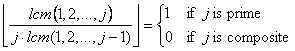His new p(n) formula is this one:which he improved as a calculating tool using the Rosen and Schoenfeld bound: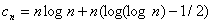obtaining:This last formula (for the prime p(200) ) is approximately 8.5 times faster than the previous one, and 65 times faster than the faster p(n) function not using the LCM characteristic function.

SMR now is investigating the following question "Which is the order of this algorithm?"

"***

On December 12, 2014, Jonathan Sondow sent the following related reference to this problem:

Sebastian Martin Ruiz and Jonathan Sondow,

Formulas for pi(n) and the nth prime, International Journal of Mathematics and Computer Science 9 (2014), no. 2, 95–98.

***Records   |  Conjectures  |  Problems  |  Puzzles